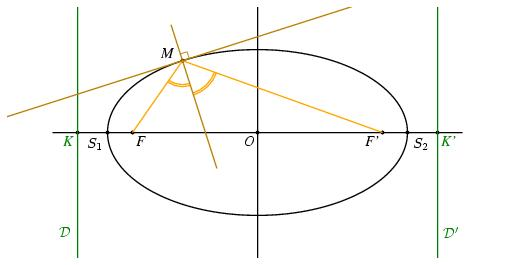# Présentation de 013.jps%% PhS

%%

50 setxunit
-5 5  setxrange
-2.5 2.5 setyrange

%%%%%%%%%%%%%%%%%%%%%%%%%%%%%%%%%%%%%%%%%%%%%%%

%% procédures

%%%%%%%%%%%%%%%%%%%%%%%%%%%%%%%%%%%%%%%%%%%%%%%

/bddroite {
8 dict begin
/kd exch def
/kg exch def
/By exch def
/Bx exch def
/B {Bx By} def
/Ay exch def
/Ax exch def
/A {Ax Ay} def
gsave
[A A B vecteur kg mulv addv A A B vecteur kd mulv addv] ligne
grestore
end
} def

%%%%%%%%%%%%%%%%%%%%%%%%%%%%%%%%%%%%%%%%%%%%%%%

%% definition d'une couleur

%%%%%%%%%%%%%%%%%%%%%%%%%%%%%%%%%%%%%%%%%%%%%%%

/coul1 {120 255 div 70 255 div 9 255 div setrgbcolor} def

%%%%%%%%%%%%%%%%%%%%%%%%%%%%%%%%%%%%%%%%%%%%%%%

%% definition des points

%%%%%%%%%%%%%%%%%%%%%%%%%%%%%%%%%%%%%%%%%%%%%%%

/a {3} def
/c {2.5} def
/b {a a mul c c mul sub sqrt} def
/e {c a div} def
/Kx {a e div} def
/K {Kx neg 0} def
/K+ {K 0 6 addv} def
/K- {K 0 -6 addv} def
/Kp {Kx 0} def
/Kp+ {Kp 0 6 addv} def
/Kp- {Kp 0 -6 addv} def
/O {0 0} def
/F {c neg 0} def
/Fp {c 0} def
/S1 {a neg 0} def
/S2 {a 0} def
/B {0 b} def

/xdet {settvar t cos a mul} def
/ydet {settvar t sin b mul} def

/Mx {120 xdet} def
/My {120 ydet} def
/M {Mx My} def
/H {M K K+ orthoproj} def
/Hp {M Kp Kp+ orthoproj} def

/bissec {F M Fp bissectrice} def
/bixx {bissec O S2 interdroite}def
/Mbixx {M bixx vecteur} def
/T {bissec M perp  Kp Kp+ interdroite} def
/MT {M T vecteur} def

/theta1 {c neg a div arccos} def
/Fcons1 {theta1 xdet theta1 ydet} def
/Fcons2 {theta1 neg xdet theta1 neg ydet} def

%%%%%%%%%%%%%%%%%%%%%%%%%%%%%%%%%%%%%%%%%%%%%%%

%% traces

%%%%%%%%%%%%%%%%%%%%%%%%%%%%%%%%%%%%%%%%%%%%%%%

/coul1 {188 255 div 128 255 div 9 255 div setrgbcolor} def

1.2 setlinewidth
[O F Fp S1 S2 K Kp M] points
[K -0.5 0 addv Kp 0.5 0 addv] ligne
[O 0 -6.5 addv O 0 6.5 addv] ligne

noir
0 360 settrange
{xdet} {ydet} courbeparam

vert
[K+ K-] ligne
[Kp+ Kp-] ligne

orange
[F M Fp] ligne

F M bixx 0.5 (=) tripointarc
bixx M Fp 0.7 (=) tripointarc

coul1
continu
M bixx -0.5 1.5 bddroite
M T -1.3 1.8 bddroite
T M bixx M vecteur M addv angledroit

%%%%%%%%%%%%%%%%%%%%%%%%%%%%%%%%%%%%%%%%%%%%%%%

%% texte

%%%%%%%%%%%%%%%%%%%%%%%%%%%%%%%%%%%%%%%%%%%%%%%

setTimesItalic
noir
(O) O [1.5 dup] dltext
(F) F [1.5 dup] drtext
(F') Fp [1.5 dup] dltext
(M) M -0.3 0.15 addv [1.5 dup] cctext

noir
<latex>
$S_1$
</latex>
S1 [1.5 dup] dltexlabel

<latex>
$S_2$
</latex>
S2 [1.5 dup] drtexlabel

vert
(K) K [1.5 dup] dltext
(K') Kp [1.5 dup] drtext

<latex>
$\mathcal{D}$
</latex>
(D) K 0 -2 addv [1.5 dup] cltexlabel

<latex>
$\mathcal{D'}$
</latex>
(D) Kp 0 -2 addv [1.5 dup] crtexlabel